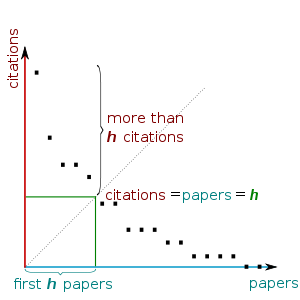0%

### leetcode 274 H-Index

Given an array of citations (each citation is a non-negative integer) of a researcher, write a function to compute the researcher's h-index.

According to the definition of h-index on Wikipedia: "A scientist has index h if h of his/her N papers have at least h citations each, and the other N − h papers have no more than h citations each."

For example, given citations = [3, 0, 6, 1, 5], which means the researcher has 5 papers in total and each of them had received 3, 0, 6, 1, 5 citations respectively. Since the researcher has 3 papers with at least 3 citations each and the remaining two with no more than 3 citations each, his h-index is 3.

Note: If there are several possible values for h, the maximum one is taken as the h-index.

Hint:

1. An easy approach is to sort the array first.
2. What are the possible values of h-index?
3. A faster approach is to use extra space.

h-index定义如下：

### 方法一C++

Python

n - i 是大于引用数citations[i]的个数。

C++

Python

## leetcode 275 H-Index II

Follow up for H-Index: What if the citations array is sorted in ascending order? Could you optimize your algorithm?

Hint:

• Expected runtime complexity is in O(log n) and the input is sorted.